Processing ......FreeComputerBooks.com Links to Free Computer, Mathematics, Technical Books all over the World

Exploring Discrete Mathematics With Maple
🌠 Top Free JavaScript Books - 100% Free or Open Source!
• Title Exploring Discrete Mathematics With Maple
• Author(s) Kenneth H. Rosen
• Publisher: Mcgraw-Hill College; 4th edition (August 1, 1996)
• Paperback 400 pages
• eBook PDF and PostScript Files
• Language: English
• ISBN-10: 0070541280
• ISBN-13: 978-0070541283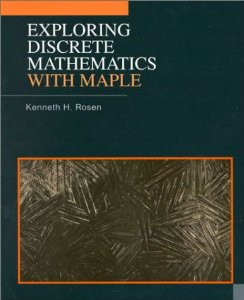Book Description

This is the first supplement in discrete mathematics to concentrate on the computational aspects of the computer algebra system Maple. Detailed instructions for the use of Maple are included in an introductory chapter and in each subsequent chapter.

Each chapter includes discussion of selected Computational and Exploration exercises in the corresponding chapter of Ken Rosen's text Discrete Math and It's Applications, Third Edition. New exercises and projects are included in each chapter to encourage further exploration of discrete mathematics using Maple. All of the Maple code in this supplement is available online via the Waterloo Maple Web site, in addition to new Maple routines that have been created which extend the current capabilities of Maple.

• N/A
Reviews, Ratings, and Recommendations: Related Book Categories: Read and Download Links:Similar Books:
•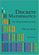Discrete Mathematics: An Open Introduction (Oscar Levin)

This is a gentle introduction to discrete mathematics. Four main topics are covered: counting, sequences, logic, and graph theory. Along the way proofs are introduced, including proofs by contradiction, proofs by induction, and combinatorial proofs.

•Mathematics for Computer Science (Eric Lehman, et al)

This book covers elementary discrete mathematics for computer science and engineering. It emphasizes mathematical definitions and proofs as well as applicable methods. explores the topics of basic combinatorics, number and graph theory, logic and proof techniques.

•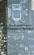Applied Discrete Structures, Fundamentals (Al Doerr, et al)

This book contains most of the fundamental concepts taught in a one semester course in discrete mathematics which is a required course for students in Computer Science, Mathematics and Information Technology.

•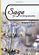Sage for Undergraduates (Gregory V. Bard)

This book is a gentle introduction to SageMath for undergraduate students toward the end of Calculus or higher-level course work such as Multivariate Calculus, Differential Equations, Linear Algebra, or Math Modeling.

•Mathematica® Programming: an Advanced Introduction

Starting from first principles, this book covers all of the foundational material needed to develop a clear understanding of the Mathematica® language, with a practical emphasis on solving problems.

•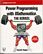Power Programming with Mathematica® (David B. Wagner)

This book unpacks Mathematica® for programmers, building insights into programming style via real world syntax, real world examples, and extensive parallels to other languages.

•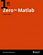Zero to MATLAB (Adam L. Lambert, PhD)

The book is structured as a tutorial rather than a reference. The MATLAB documentation is extensive and so my focus will be to outline basic algorithmic thinking and MATLAB syntax with explicit examples.

•Scientific Computing with MATLAB (Paul Gribble)

The goal of this book is to provide you with skills in scientific computing: tools and techniques that you can use in your own scientific research. We will focus on learning to think about experiments and data in a computational framework.

•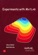Experiments with MATLAB (Cleve Moler)

This book provides an excellent initiation into programming in MATLAB while serving as a teaser for more advanced topics. It provides a structured entry into MATLAB programming through well designed exercises.

Book Categories
 :All CategoriesTop Free BooksRecent BooksMiscellaneous BooksComputer EngineeringComputer LanguagesComputer ScienceData Science/DatabasesJava and Java EE (J2EE)Linux and UnixMathematicsMicrosoft and .NETMobile ComputingNetworking and CommunicationsSoftware EngineeringSpecial TopicsWeb Programming
Other Categories